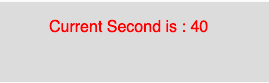Open In App

# p5.js second() function

The second() function in p5.js is used to return the current second of the system clock. The value of second() function lies between 0 to 59.

Syntax:

`second()`

Parameters: The function does not accept any parameter.

Return Value: This function returns an integer value which represents the current second.

Below program illustrates the second() function in p5.js:

Example: This example uses second() function to return the current second.

 `function` `setup() {`` ` `    ``// Create Canvas of size 270*80``    ``createCanvas(270, 80);``}`` ` `function` `draw() {``     ` `    ``// Set the background color``    ``background(220);``     ` `    ``// Store value of second() function``    ``// to the variable``    ``let s = second();``     ` `    ``// Set the font size``    ``textSize(16);``     ` `    ``// Set the font color``    ``fill(color(``'red'``));``     ` `    ``// Display result ``    ``text(``"Current Second is : "` `+ s, 50, 30);``}`

Output:Reference: https://p5js.org/reference/#/p5/second